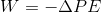# SAT II Physics : Electric Potential Energy

## Example Questions

### Example Question #1 : Electric Fields

On which of the following does the amount of work required to move a charge in an electric field depend?

Only the path traveled

Neither the potential nor the path traveled

Both the potential and the path traveled

Only the magnetic field

Only the change in potential

Only the change in potential

Explanation:

Work done by electric field is defined:Notice that the only variable in the equation is the potential, so this is the only quantity on which work depends.

### All SAT II Physics Resources